## Main

The situation we have in mind is shown in Fig. 1. A quantum system composed of N particles (such as qubits) is organized in space according to a particular geometry (in the figure, a one-dimensional lattice). Neighbouring systems are coupled to some local environments, which are dissipative in nature and tend to drive the system to a steady state. Our idea is to engineer those couplings, so that the environments drive the system to a desired final state. The coupling to the environment will be static, so that the desired state is obtained after some time without having to actively control the system. Note that the role of the environments is to dissipate (or, more precisely, evacuate) the entropy of the system, and by choosing the couplings appropriately we can use this effect to drive our system.

We will show first how to design the interactions with the environment to implement universal quantum computation. This new method, which we refer to as dissipative quantum computation (DQC), defies some of the standard criteria for quantum computation because it requires neither state preparation, nor unitary dynamics6. However, it is nevertheless as powerful as standard quantum computation. Then we will show that dissipation can be engineered7 to prepare ground states of frustration-free Hamiltonians. Those include matrix product states4,8,9 (MPSs) and projected entangled pair states5,9 (PEPSs), such as graph states10 and Kitaev11 and Levin–Wen12 topological codes. Both DQC and dissipative state engineering (DSE) are robust in the sense that, given the dissipative nature of the process, the system is driven towards its steady state independent of the initial state and hence of eventual perturbations along the way.

Here, we will concentrate first on DQC, showing how given any quantum circuit one can construct a locally acting master equation for which the steady state is unique, encodes the outcome of the circuit and is reached in polynomial time (with respect to the one corresponding to the circuit). Then we will show how to construct dissipative processes that drive the system to the ground state of any frustration-free Hamiltonian. In the Methods section, we will prove that MPS (ref. 9) and certain kinds of PEPS (ref. 9) can be efficiently prepared using this method, and in Supplementary Information we will give details of the proofs. In this letter we will not consider specific physical set-ups where our ideas can be implemented. Nevertheless, the Methods section will provide a universal way of engineering the master equations required for DQC and DSE, which can be easily adapted to current experiments 13 based on, for example, atoms in optical lattices 14 or trapped ions15. Thus, we expect that our predictions may be experimentally tested in the near future.

Let us start with DQC by considering N qubits in a line and a quantum circuit specified by a sequence of nearest-neighbour qubit operations {Ut}t=1T. We define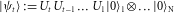, so that |ψT〉 is the final state after the computation. Our goal is to find a master equation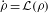with a Liouvillian in Lindblad form16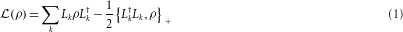where the Lkacts locally and has a steady state, ρ0: (1) that is unique; (2) that can be reached in a time poly(T); (3) such that ψT can be extracted from it in a time poly(T). As in Feynman’s construction of a quantum simulator3, we consider another auxiliary register with states {|t〉}t=0T, which will represent the time. We choose the Lindblad operators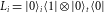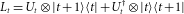where i=1,…,N and t=0,…,T. It is clear that the L terms act locally except for the interaction with the extra register, which can be made local as well. Furthermore,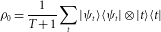is a steady state, that is,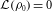. Given such a state, the result of the actual quantum computation can be read out with probability 1/T by measuring the time register. In Supplementary Information, we show that ρ0 is the unique steady state and that the Liouvillian has a spectral gap Δ=π2/(2T+3)2. This means indeed that the steady state will be reached in polynomial time in T. Note that this gap is independent of N as well as of the actual quantum computation that is carried out (that is, independent of the Ut). It is also shown that the same gap is retained if the clock register is encoded in the unary way proposed by Kitaev and co-workers17, making the Lindblad operators strictly local. A sketch of the proof is as follows. First, we do a similarity transformation on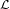that replaces all gates Ui with the identity gates, showing that its spectrum is independent of the actual quantum computation. Second, another similarity transformation is done that makesHermitian and block-diagonal. Each block can then be diagonalized exactly leading to the claimed gap.

In some sense, the present formalism can be seen as a robust way of doing adiabatic quantum computation18 (errors do not accumulate and the path does not have to be engineered carefully) and implementing quantum random walks19, and it might therefore be easier to tackle interesting open questions, such as the quantum probabilistically-checkable-proofs theorem, in this setting20. In addition, it seems that the dissipative way of preparing ground states is more natural than to use adiabatic time evolution, as nature itself prepares them by cooling.

Let us now turn to DSE and consider again a quantum system with N particles on a lattice in any dimension. We are interested in ground states Ψ, of Hamiltoniansthat are frustration-free, meaning that Ψ minimizes the energy of each Hλ individually, and local in the sense that Hλ acts non-trivially only on a small set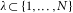of sites (for example, nearest neighbours). We can assume the terms Hλ to be projectors and we will denote the orthogonal projectors by Pλ=1Hλ. States Ψ of the considered form are, for example, all PEPS (including MPS and stabilizer states21).

We will consider discrete time evolution generated by a trace-preserving completely positive map instead of a master equation. These two approaches are basically equivalent22 as every local completely positive map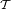can be associated with a local Liouvillian through, which leads to the same fixed points and spectrum. We choose completely positive maps of the form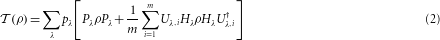where the pλterms are probabilities and Uλ,1,…,Uλ,m is a set of unitaries acting non-trivially only within region λ. They effectively rotate part of the high-energy space (with support of Hλ) to the zero-energy space, so that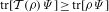increases. As for Liouvillians (1), we could similarly take Lλ,i=UiHλ, or the ones associated with the completely positive map.

We show now that for every frustration-free Hamiltonian, the completely positive map in equation (2) converges to the ground-state space if we choose the unitaries Uλ,i to be completely depolarizing, that is,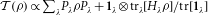. For ease of notation, we will explain the proof for the case of a one-dimensional ring with nearest-neighbour interactions labelled by the first site λ=1,…,N. Assume ρ is such that its expectation value with respect to the projector Ψ onto the ground-state space of H is non-increasing under applications of, that is, in particular. Expressing this in the Heisenberg picture in which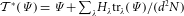, we get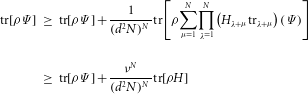where the first inequality comes from discarding (positive) terms in the sum and the second one is due to bounding all partial traces of Hλ from below by the respective smallest eigenvalue ν. Note that the latter is strictly positive unless H has a product state as the ground state (in which case the statement becomes trivial). Hence, we must have tr[ρ H]=0; that is, ρ is a ground state of H. It is easily seen that the same argument applies for more general interactions on arbitrary lattices.

Once we have shown that the steady state after the application of the completely positive map lies within the desired subspace (the ground-state space of the frustration-free Hamiltonian), the next question to be addressed is how efficient the process is. This depends on the spectral gap, δ, of the completely positive map (or, equivalently, of the corresponding Liouvillian), as the time to reach the steady state,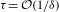. Thus, the above procedure will be efficient as long as the gap vanishes only polynomially with the number of systems, N. Similarly to what occurs with many-body Hamiltonians, the determination of such a gap is, in general, very complicated. For a wide range of interesting models, however, it can be proved that this gap scales favourably. This is the case for all MPS as well as for a rich subfamily of PEPS that includes all stabilizer states (such as Kitaev’s toric code11 and the Levin–Wen states12). In the Methods section, we characterize such a subfamily of states, and in Supplementary Information we give the technical proofs of our statements. Here, we will qualitatively explain how our method works efficiently for some families of states. For that we note that the action of the completely positive map (2) can be interpreted as randomly choosing a region λ (according to pλ, which we may set equal to 1/N), then measuring Pλ and applying a correction according to the unitaries if the outcome was negative. We denote by Rn the set of regions λ where ϕ satisfies the condition Hλ|ϕ〉=0. If we measure now in one of those regions, we will obviously obtain a positive result, and thus Rn will remain the same. If we measure in another region, we may have a positive or negative result, something that may change the set Rn. By imposing certain conditions on the operators Hλ and Uλ,i, we can make sure that in each step Rn cannot be reduced and that the probability of being enlarged is non-vanishing. This automatically ensures that the τ scales only polynomially with the number of systems. In one dimension, however, one can get rid of all those restrictions and show that any MPS can be prepared in a time that also scales favourably with N. The fact that all MPS states can be prepared with our method, together with the results reported in refs 23, 24, automatically implies the existence of phase transitions driven by dissipation in the following sense. By changing the parameters of the operators Hλ appearing in the completely positive map (2), we change the steady state of that map. It is possible to choose models for which that state changes abruptly at some particular value of that parameter in such a way that the correlation length diverges and an order parameter appears (an example can be found in the Supplementary Information).

We have investigated the computational power of purely dissipative processes, and proved that it is equivalent to that of the quantum circuit model of quantum computation. We have also shown that dissipative dynamics can be used to create ground states (such as MPS or PEPS) of frustration-free Hamiltonians of strongly correlated quantum spin systems. We believe that these new methods can be experimentally tested using atoms or ions with current set-ups (see the Methods section).

Let us stress that we have been concerned here with a proof-of-principle demonstration that dissipation provides us with an alternative way of carrying out quantum computations or state engineering. We believe, however, that much more efficient and practical schemes can be developed and adapted to specific implementations. We also think that these results open up some interesting questions that deserve further investigation: for example, how the use of fault-tolerant computations can make our scheme more robust, or how one can design translationally invariant completely positive maps that prepare MPS more efficiently, or the importance and generality of the set of commuting Hamiltonians (see the Methods section), which is intimately connected to the fixed points of the renormalization group transformations on PEPS (as it happens with MPS; ref. 25). Furthermore, the model of DQC might well lead to the construction of new quantum algorithms, as, for example, quantum random walks can more easily be formulated within this context. Finally, other ideas related to this work can be easily addressed using the methods introduced; for example, thermal states of commuting Hamiltonians can be engineered using DSE because the Metropolis way of sampling over classical spin configurations can be adopted to the case of commuting operators. Similar techniques could be applied to free fermionic and bosonic systems, and, more generally, it should be possible to devise DSE schemes converging to the ground or thermal states of frustrated Hamiltonians by combining unitary and dissipative dynamics.

Note added. Concurrently with the submission of this paper, refs 26 and 27 appeared in which a similar quantum-reservoir engineering was used to prepare many-body states and non-equilibrium quantum phases.

## Methods

### Engineering dissipation.

Here we show how to engineer the local dissipation that gives rise to the master equations (1) and completely positive maps (2). They are composed of local terms, involving few particles (typically two), so that we just have to show how to implement those. To simplify the exposition, we will treat those particles as a single one and assume that one has full control over its dynamics (for example, one can apply arbitrary gates).

Let us start with the completely positive maps. It is clear that by applying a quantum gate to the particle and a ‘fresh’ ancilla and then tracing the ancilla one can generate any physical action (that is, completely positive map) on the system. Furthermore, by repeating the same process with short time intervals one can subject the system to an arbitrary time-independent master equation. This last process may not be efficient. An alternative way works as follows. Let us assume that the ancilla is a qubit interacting with a reservoir such that it fulfils a master equation with Liouville operator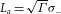, where σ=|0〉〈1|. Now, we couple the ancilla to the system with a Hamiltonian H=Ω(σL+σL). In the limit ΓΩ, one can adiabatically eliminate the level |1〉 of the ancilla28 by applying second-order perturbation theory to the Liouvillian (albeit for non-Hermitian operators). In this way we obtain an effective master equation for ρ describing the system alone, with Liouville operator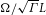. By using several ancillas with Hamiltonians H=Ω(σLi+σLi) and following the same procedure we obtain the desired master equation. Although we have not specified here a physical system, one could use atoms. In that case, the ancilla could be an atom itself with |0〉 and |1〉 an electronic ground and excited level, respectively, so that spontaneous emission gives rise to the dissipation. The coupling to the system (other atoms) could be achieved using standard ideas used in the implementation of quantum computation using those systems13.

### Efficient state preparation.

We have shown that it is possible to engineer dissipative processes that prepare ground states of frustration-free Hamiltonians in steady state. In the proof, the time for this preparation scales as NN, which may be an issue for experiments with large number of particles. Here we give much more efficient methods for certain classes of frustration-free Hamiltonians.

We consider first frustration-free Hamiltonians for which [Hλ,Hμ]=0 and show that, under certain conditions, the corresponding ground states can be prepared in a time that scales only polynomially with the number of particles. The corresponding set of ground states contains important families, such as stabilizer states (for example, cluster states and topological codes), or certain kinds of PEPS, namely, those that have (commuting) parent Hamiltonians with the injectivity condition (as defined in refs 8, 29). Note that there was no known way of efficient preparation for the latter.

Loosely speaking, we will consider two classes of Hamiltonians. (1) Hamiltonians for which all excitations can be locally annihilated. In this case the time of convergence scales as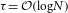. (2) Interactions where excitations have to be moved along the lattice before they can annihilate and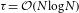.

To see how the first case can occur notice that, when iterating, the correction on λ does not change the outcome of previous measurements on neighbouring regions because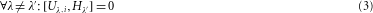In fact, this can always be achieved by regrouping the regions into larger ones having an interior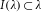on which only Hλacts non-trivially and letting the Uλ,i solely act on I(λ). Denote by q the largest probability for obtaining twice a negative measurement outcome on the same region λ. The energyafter M applications ofdecreases then as N(1−(1−q)/N)M such that it takes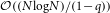steps to converge to a ground state. The relaxation time of the corresponding Liouvillian is thus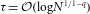. Clearly, this is a reasonable bound only if q<1, a condition possibly incompatible with equation (3).

Note that for all stabilizer states we can achieve q=0, because there exists always a local unitary (acting on a single qubit) so that HλUλHλ=0. A class of stabilizer states where this is compatible with equation (3) are the so-called graph states10. In this case, λ labels (with some abuse of notation) a vertex of a graph and, where σ(λ)is a Pauli operator acting on site λ and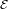is the set of edges of the graph. Obviously, Uλ=σz(λ) does the job. In this special case, we can get even faster convergence when using the Liouvillian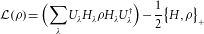The corresponding relaxation time can be determined exactly by realizing that the spectrum ofequals that of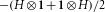so that τ=1 (see Supplementary Information).

For the second type of commuting Hamiltonians, equation (3) and q<1 are incompatible. However, we can still prove fast convergence by relaxing equation (3) such that within each region λ the Uλ acts on a site closest to a predetermined site (say the origin) on the lattice and thus commutes with all terms Hλ that are further away (see Supplementary Information for details). In this way excitations are moved over the lattice before they can annihilate. As this requires extra time proportional to the system’s size, we get.

We turn now to another family of ground states of frustration-free Hamiltonians, namely MPS (ref. 9). For the sake of clearness, we will consider here translationally invariant Hamiltonians, although the analysis can be straightforwardly extended to systems without that symmetry. We will specify a completely positive map to prepare states of the form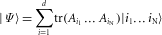where the A terms are D×D matrices. We assume the injectivity property29, which implies that Ψ is the unique ground state of a nearest-neighbour frustration-free ‘parent’ Hamiltonian that has a gap. Denoting by ρ the reduced density operator corresponding to particles k and k+1, Hk and Pk=1−Hk will denote the projectors onto its kernel and range, respectively. Note that tr(Pk)=D2. We take N=2n for simplicity, but this is clearly not necessary. We construct the channelin several steps. We first define a channel acting on two neighbouring particles k,k+1, as follows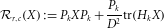Here, k=2r−1(2c−1), where r=1,…,n and c=1,…,2nr. The action of these maps has a tree structure, where the index rindicates the row in the tree, whereas c does it for the column. Now we define recursively,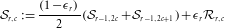Here, r=2,…,n, c=1,…,2nr,and εr+1=1/Mr, where M=C N2 and C1 (see Supplementary Information). Note that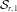acts on the first 2r particles,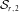on the next 2r and so on. We finally defineIn the Supplementary Information, we show that this map achieves the fixed point (up to an exponentially small error in C) in a time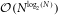. The intuition behind the completely positive map (4) is that the channels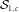, which are the ones that most often applied, project the state of every second nearest neighbour onto the right subspace. Then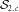do the same with half of the pairs that have not been projected. Then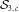does the same on half of the rest, and so on.